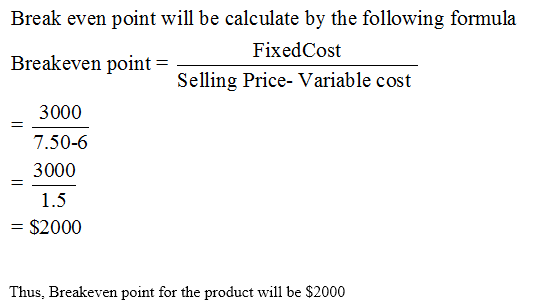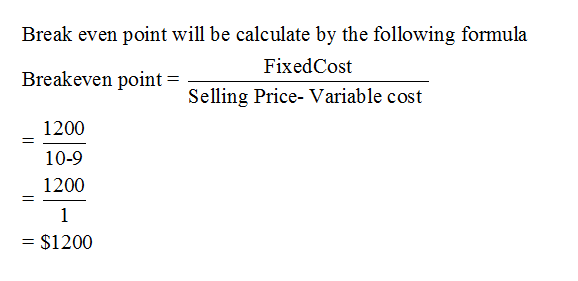# North Pole Toys needs to decide on their newest product line for Christmas. They narrowed their options to two possibilities: Product A would incur a fixed cost of \$3,000 and a variable cost of \$6 per unit and sells for \$7.50; Product B would incur a fixed cost of \$1,200 and a variable cost of \$9 per unit and sells for \$10.__________4a. What is the breakeven point for each of the two products?__________4b. What is the point of indifference between the two products?

Question
2 views

North Pole Toys needs to decide on their newest product line for Christmas. They narrowed their options to two possibilities: Product A would incur a fixed cost of \$3,000 and a variable cost of \$6 per unit and sells for \$7.50; Product B would incur a fixed cost of \$1,200 and a variable cost of \$9 per unit and sells for \$10.

__________4a. What is the breakeven point for each of the two products?

__________4b. What is the point of indifference between the two products?

check_circle

Step 1

For the product A

Given:

Fixed Cost= \$3000

Variable cost= \$6

Selling Price= \$7.50Step 2

For the product B

Given:

Fixed Cost= \$1200

Variable cost= \$9

Selling Price= \$10...

### Want to see the full answer?

See Solution

#### Want to see this answer and more?

Solutions are written by subject experts who are available 24/7. Questions are typically answered within 1 hour.*

See Solution
*Response times may vary by subject and question.
Tagged in